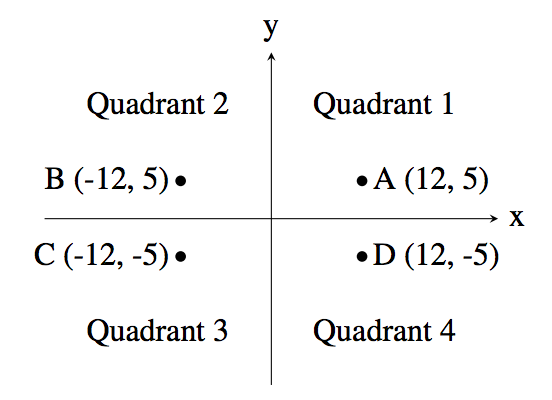시간 제한 메모리 제한 제출 정답 맞은 사람 정답 비율
1 초 512 MB 428 402 385 93.902%

## 문제

A common problem in mathematics is to determine which quadrant a given point lies in. There are four quadrants, numbered from 1 to 4, as shown in the diagram below:For example, the point A, which is at coordinates (12, 5) lies in quadrant 1 since both its x and y values are positive, and point B lies in quadrant 2 since its x value is negative and its y value is positive.

Your job is to take a point and determine the quadrant it is in. You can assume that neither of the two coordinates will be 0.

## 입력

The first line of input contains the integer x (−1000 ≤ x ≤ 1000; x ≠ 0). The second line of input contains the integer y (−1000 ≤ y ≤ 1000; y ≠ 0).

## 출력

Output the quadrant number (1, 2, 3 or 4) for the point (x, y).

## 예제 입력 1

12
5


## 예제 출력 1

1


## 예제 입력 2

9
-13


## 예제 출력 2

4